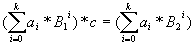# Problem H

Base equality

## Source code: base.*

Numerous are the moments I as a programmer have been frustrated by the tedious conversions between decimal numbers and hexadecimal ones. Why have we chosen 10 as a base in our everyday numerical presentations, when 16 seems so practically appealing? Obviously because everyone is not the computer geek I am. Maybe some day the world will fully realise the benefits of the hexadecimal system. In the meantime I have to learn to master the base conversions since most of the time numbers do not resemble one another in different bases.

Sometimes peculiar relationships emerge among the different base representations of numbers though. For instance, I noticed just the other day that 104010 * 4 = 104016, i.e. (1*103+0*102+4*101+0*100)*4=(1*163+0*162+4*161+0*160). It made me wonder how often this is the case, that is, the digits of a number in one base, are exactly the same as the digits of a multiple of the number in another base. Formally, let B1<B2 be positive integers, and a0,a1,…,ak be integers in [0…B1-1]. For which ai’s is there a positive integer c such that### Input

On the first line of input is a positive integer n telling the number of test cases that follow. Each test case is on a line of its own and consists of two integer bases B1, B2, 9<B1<B2<100, and two integer range elements, r1, and r2, 0<r1<r2<10000. Notice that all numbers in the input are given in the base 10.

### Output

For each test case, there should be one row containing the largest integer i, fulfilling r1<i<r2, for which there is a positive integer c such that the digits of i in the base B1, are exactly the same as the digits of i*c in the base B2. If no such integer i exists, output the text ‘Non-existent.’.

Example input:              Example output:

4                           1040

10 16 1 2000                4240

10 16 1 4999                Non-existent.

10 14 10 9999               9240

11 14 10 9999